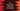# Find the cube root of a number in C++# Introduction :

cbrt function is used to find out the cube root of a number in C++. In this tutorial, we will learn how to use cbrt function with an example.

## cbrt function :

cbrt is used to find the cube root of a number in C++. This is defined in cmath header file.

## Definition :

As per C++ 11 specification, cbrt is defined as below :

``````double cbrt (double x);
float cbrt (float x);
long double cbrt (long double x);
double cbrt (T x); ``````

The last one is for integral type. This overloaded method casts the integral type to double.

## Parameter and Return value :

This method takes a parameter of type double, float, long double, or integral type.

It returns the cubic root of the parameter.

## Example :

In this example, we will take the number as input from the user.

``````#include <iostream>
#include <cmath>
using namespace std;
int main()
{
int n;
cout << "Enter a number to find out the cubic root : " << endl;
cin >> n;
cout << "The cubic root is : " << cbrt(n) << endl;
return 0;
}``````

## Sample Output :

``````Enter a number to find out the cubic root :
27
The cubic root is : 3``````